Important Relationships Between Properties Of Soil

There are few important relationships between properties of soil that will help us solve many soil mechanics related problems.

Porosity and Void Ratio :

We define void ratio as volume of voids to the volume of solids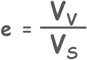and porosity as volume of voids to the total volume of soil mass.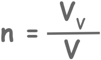Using 3 phase diagram of soil, in the void ratio equation we can write Vs, which is volume of solids, as total volume minus volume of voids.Then divide both numerator and denominator with total volume of soil mass capital V.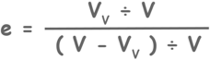now we can see the porosity in the equation.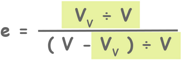Hence the relationship between void ratio and porosity is established.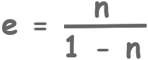Which can also be written as this.Now lets develop relationship between void ratio, water content, specific gravity and degree of saturation

We write void ratio as volume of voids to the volume of solidslets multiply and divide this equation with volume of water and rearrange a little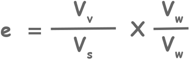Now we can write volume of water as weight of water to the unit weight of water, and similarly volume of solids as weight of solids to the unit weight of solids.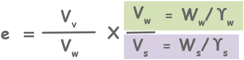After slight rearrangement we can write the unit weight of solids as specific gravity of solids multiply with unit weight of water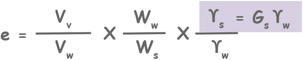Again after re-arranging the equation we can see that volume of water divided by volume of voids is degree of saturation and weight of water divided by weight of solids is water content.So after rewriting the equation we found the relationship as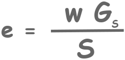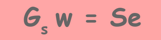Now we will establish an important relationship between unit weight, specific gravity, void ratio, water content and unit weight of water.

We know bulk unit weight gamma T is written as total weight of soil sample to its volume.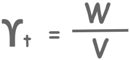Total weight of any soil is actually weight of solids and weight of water present in it and its volume combines volume of solids and volume of voids.Take out W s in numerator and Vs in denominator.Now here we notice that water content and void ratio in the equation.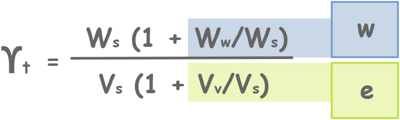also Ws divided by Vs in the equation is unit weight of solids which we write as specific gravity times unit weight of water.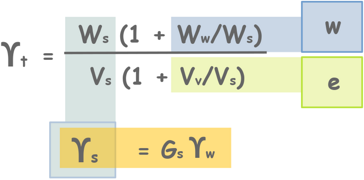So by substituting these, we get this.And now here we know

Gsw = Se

So we can write water content w in the equation as thisand after substituting we get a new relationship to be remembered.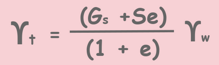Using this relationship we can also see that…

When soil is dry, bulk unit weight becomes dry unit weight and degree of saturation of soil becomes zero as no water is present in it. Then equation will become something like this: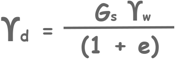Using this equation we can find the dry unit weight of soil if other parameters are known.

On the other hand, when soil is fully saturated then degree of saturation will become one and equation will look like this.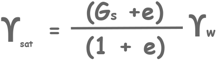Now as we know submerged unit weight of a soil, gamma dash, is equal to gamma sat minus gamma w

ϒ' = ϒsat - ϒw

now using previously established equation we can write gamma sat as this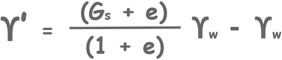and by simplifying it, we get a new relationship.Lets establish one more relationship between bulk unit weight, dry unit weight and water content

bulk unit weight gamma T is total weight divided by its volume.And total weight can be written as weight of solids plus weight of water.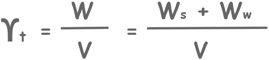We can take out weight of solids from the numerator and we can see in the equation dry unit weight and water content.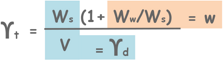Which forms the following relationship.We can establish many such relationships but these are most important and frequently used equations in soil mechanics problems

Tags : relationship

Published on :2019-08-04